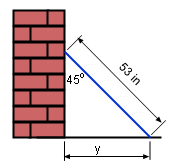Ch 2. Derivatives I Multimedia Engineering Math DerivativeFormulas TrigonometricFunctions Differentiability ChainRule ImplicitDifferentiation
 Chapter 1. Limits 2. Derivatives I 3. Derivatives II 4. Mean Value 5. Curve Sketching 6. Integrals 7. Inverse Functions 8. Integration Tech. 9. Integrate App. 10. Parametric Eqs. 11. Polar Coord. 12. Series Appendix Basic Math Units Search eBooks Dynamics Fluids Math Mechanics Statics Thermodynamics Author(s): Hengzhong Wen Chean Chin Ngo Meirong Huang Kurt Gramoll ©Kurt GramollMATHEMATICS - CASE STUDY IntroductionTilt Ice Hockey Stick After a game, Alan put his ice hockey stick against a vertical wall, but the bottom of the stick slid away from the wall. He wonders how fast the slipped distance changes with respect to the angle made by the wall and the stick. What is known: The length of the stick is 53 in. The angle made by the wall and the stick is θ = 45o. The slipped distance is y. Questions How fast does the slipped distance change with respect to the angle that is made by the wall and the stick is 45o or π/4? Approach Derivative with respect to angle can be used in this case to calculate the rate of slippage.